Zth-measurement (SiC) - Zth Messtechnik - transient thermal impedance of power semiconductors - thermal-electrical simulationYour partner for thermal impedance measurement of power semiconductors
Zth-Messtechnik Kiffe
Go to content

Zth-measurement (SiC)

Example
In power electronics, there is an increasing trend towards silicon carbide (SiC) as semiconductor material. Silicon carbide power semiconductors have thanks to
superior material properties compared to silicon several advantages such as lower switching losses, higher switching speeds, better thermal conductivity and
a higher breakdown field strength.

The following example shows a Zth-measurement on a SiC Power MOSFET.

Example: Zth-measurement on a 90A/1200V SiC Power MOSFET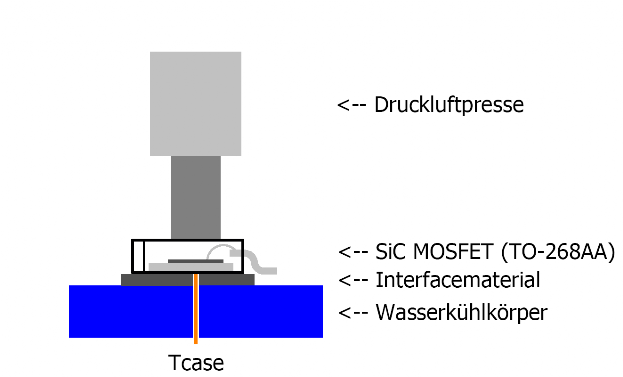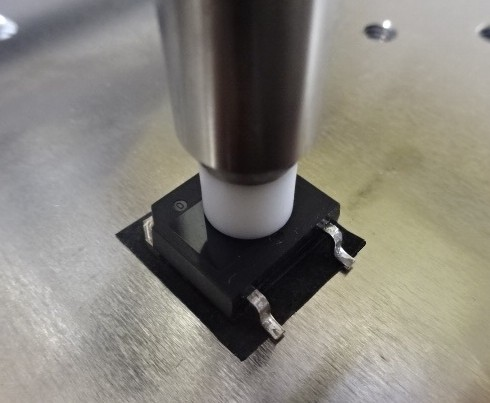Zth control protocol (40A Zth measuring station)

Date: 28.01.2019  time: 14:11:51

Zth-measurement on a 90A/1200V SiC Power MOSFET

Heating: Body-Diode
Measure up: Body-Diode

Heat sink: closed water cooler
Thermal compound: Graphite filled high performance silicone film,
thickness: 200µm
Cooling medium: domestic cooling water

File: 90A-SiC-MOSFET.dat

Heating up sec: 30sec     cooling down sec: 30sec

Pv = 100,011W    Uload = 4,050V    Iload = 24,70A

Imeas = 0,04568A    Ug = -10,006V

Cooling down phase:
Channel 5: contact pressure = 99,86N
Channel 6: water flow = 15,25l/min
Almemo: Tcase = 6,40°C

Heating up phase:
Channel 5: contact pressure = 99,91N
Channel 6: water flow = 15,25l/min
Almemo: Tcase = 23,17°C

Calibration value: T = 6,40°C     U = 2,6532V
Calibration value: T = 31,53°C   U = 2,5931V
Calibration value: T = 56,67°C   U = 2,5357V
Zth evaluation protocol

Date: 28.01.2019  time: 17:21:19

Curve from file: 90A-SiC-MOSFET.dat

Voltage/temperature conversion:
Table of file: 90A-SiC-MOSFET.pk1

Thermal power loss (thermal equilibrium) = 100,011W

Ta(meas curve) = 6,37°C                    Ta(eval curve) = 6,37°C
Tj(meas curve) =  54,04°C                  Tj(eval curve) = 54,10°C

Rthja(meas curve) =  0,476703K/W      Rthja(eval curve) = 0,477240K/W
Rthca(meas curve) = 0,167682K/W      Rthca(eval curve) = 0,167682K/W
Rthjc(meas curve) =  0,309022K/W      Rthjc(eval curve) =  0,309559K/W

First measurement point = 9,5400e-06s,   last meas. point = 3,0000e01s

Partial fraction network (Foster):
Rj, Cj, and Tau values from t(0)
Rj (K/W)            Cj (Ws/K)           Tau (s)
0,005112101      0,017681602      0,000090390
0,058032216      0,016462422      0,000955351
0,063596560      0,061387473      0,003904032
0,107753821      0,224079046      0,024145373
0,115536616      0,966232553      0,111635240
0,102589523      4,609384487      0,472874558
0,023241496      92,38472272      2,147159208
0,001378006      8363,157546      11,52447732

equivalent continues fraction network (Cauer):
Rj, Cj, and Tau values from t(0)
Rj (K/W)            Cj (Ws/K)           Tau (s)
0,027981337      0,007177932      0,000200848
0,081157047      0,007010224      0,000568929
0,076992853      0,057176949      0,004402216
0,118928719      0,178525533      0,021231813
0,102076545      1,043926870      0,106560448
0,056742233      7,099203403      0,402824655
0,012545742      162,8499781      2,043073737
0,000815863      13914,44479      11,35228689
Log file generated by the control program 90A-SiC-MOSFET.pk1                                     Log file generated by the evaluation program 90A-SiC-MOSFET.pk2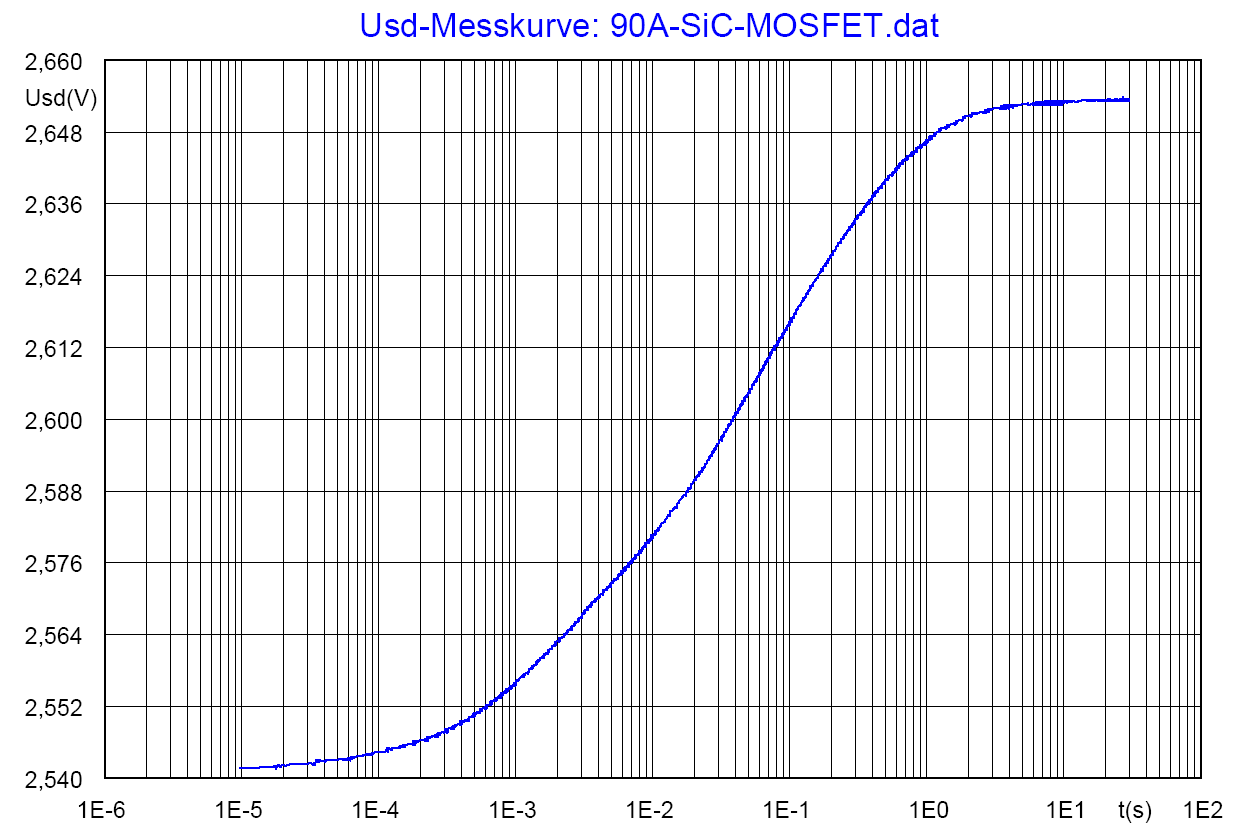The recorded Usd curve after switching off the load current at a measuring current of 46mA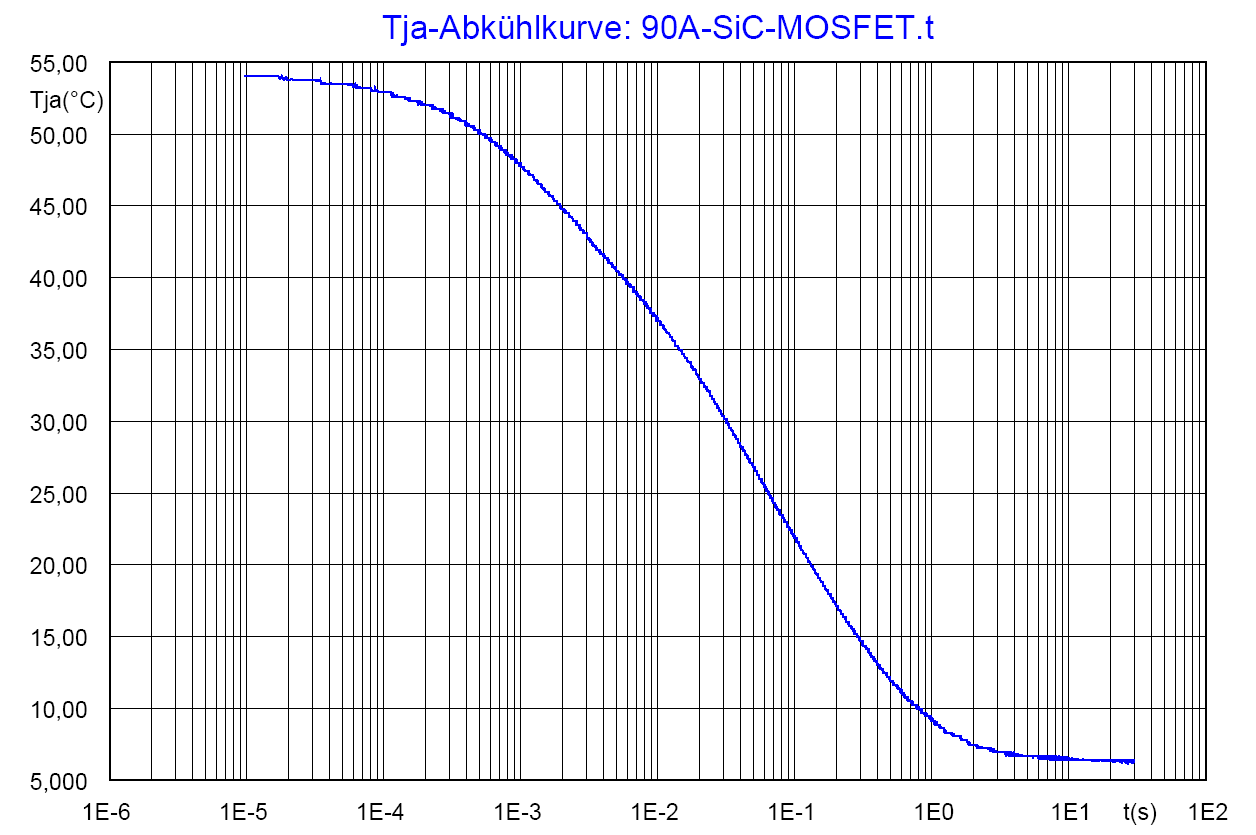From the Usd curve and the calibration values (file 90A-SiC-MOSFET.pk1) converted T(junction ambient) cooling curve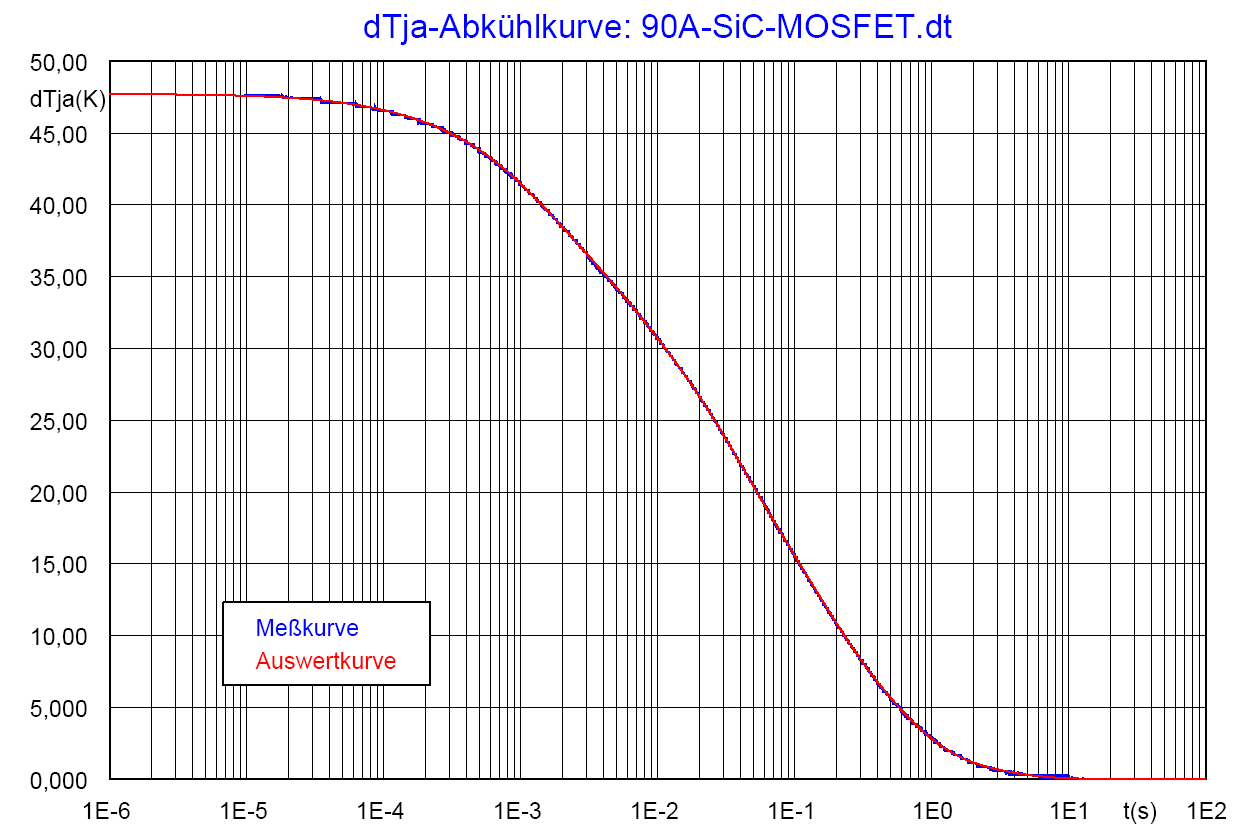Measured cooling curve ΔTja (measured curve blue) in comparison to the evaluation result (evaluation curve red) from the partial fraction network table
(file 90A-SiC-MOSFET.pk2)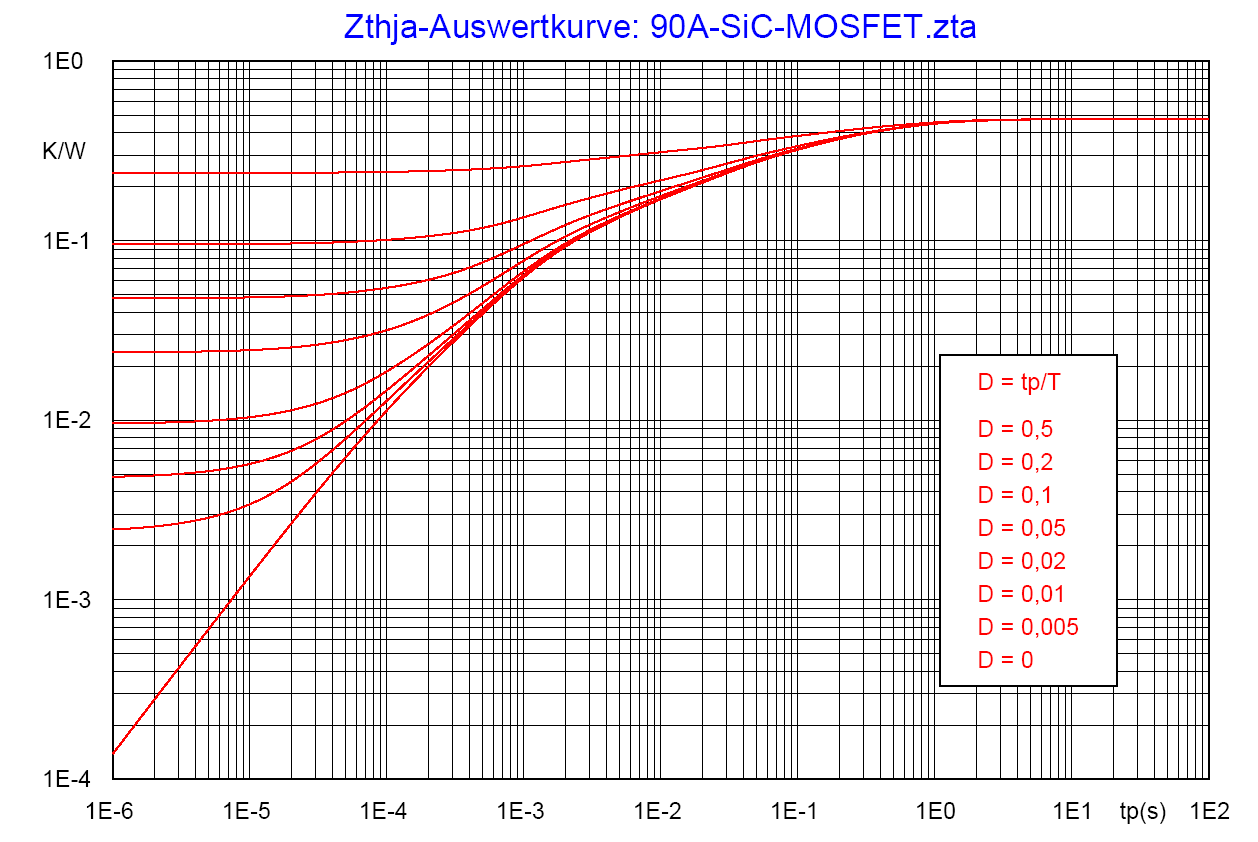Zth-diagram: Zth=f(tp), parameter D=tp/TBack to content